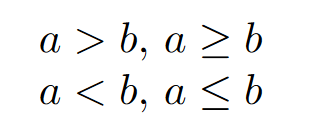How To Write The Less Than Symbol In LaTeX?

# How To Write The Less Than Symbol In LaTeX?

This post may contain affiliate links that allow us to earn a commission at no expense to you. Learn more

This article aims to show you the simplest and easiest way to write the less than symbol in LaTeX.

The less than symbol is part of the inequalities symbols you are familiar with, used in everyday life. That is why today you will learn more about this particular one, and how to write this symbol in your LaTeX document.

## Inequality symbols

In mathematics, by definition an inequality is a relation that makes a non-equal comparison between two values, numbers, or expressions. To represent an inequality we used the inequality symbols, there are  different kinds of inequalities, for example,Left to right: greater than, greater than or equal, less than, and less than or equal

Today we center our attention on the less than a symbol. This symbol or sign denoted by “<”, states that the first number is less than the second one.

The less than inequality is known as a strict inequality, meaning that 1 is strictly less than 3.

## Less than symbol in LaTeX

If you know your keyboard you know where the key “<” is, you could be tempted to use it, but sooner you would see that the output does not look like what you expected. Therefore this symbol cannot be used in text mode.

In LaTeX to write the “<” symbol, you only can use it inside math mode -denoted by a pair of dollar signs- in the latex code. The complete command would look like $<$, let’s see an example

\documentclass{article}
\begin{document}
Less than command \verb|$<$|: $<$ \\
$100 < 101$ \\
\end{document}


Going the extra mile, in case you are wondering how to write the less than or equal symbol -this is known as a not strictly inequality- you use the command \leq inside math mode, alongside the amssymb package.

\documentclass{article}
\begin{document}
Less than or equal \verb|\leq|: $\leq$ \\
$a \leq b$
\end{document}


There you have it, the LaTeX commands for the less than, and the less than or equal symbols.

Another thing to notice is that there could be more combinations that include both of these symbols but they are not so common.

I hope this tutorial was helpful, and as always keep writing in LaTeX

All images were created in LaTeX by the Author.